## Point-Line Distance--2-D

Given a lineand a point), in slope-intercept form, the equation of the line is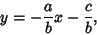(1)

so the line has Slope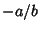. Points on the line have the vector coordinates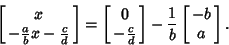(2)

Therefore, the Vector(3)

is Parallel to the line, and the Vector(4)

is Perpendicular to it. Now, a Vector from the point to the line is given by(5)

Projecting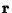onto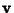,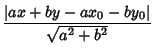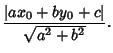(6)

If the line is represented by the endpoints of a Vectorand, then the Perpendicular Vector is(7)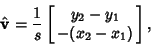(8)

where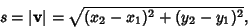(9)

so the distance is(10)

The distance from a point (,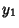) to the linecan be computed using Vector algebra. Let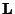be a Vector in the same direction as the line(11)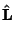(12)

A given point on the line is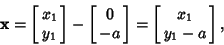(13)

so the point-line distance is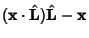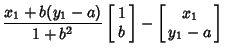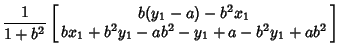(14)

Therefore,(15)

This result can also be obtained much more simply by noting that the Perpendicular distance is just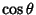times the vertical distance. But the Slope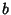is just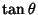, so(16)

and(17)

The Perpendicular distance is then(18)

the same result as before.